# Electro-Absorption Modulator

## Introduction

For telecom applications, high-speed optical modulators with small size & low operating voltage are highly desired. The electro-absorption modulator (EAM) is one technology commonly used to achieve these goals. Compared to an electro-optic modulator, an EAM can operate with much lower voltages (only a few volts), and can operate with a modulation bandwidth ~50 Ghz. In addition, an EAM can be integrated with a laser diode onto a single chip to form a photonic integrated circuit (PIC) data transmitter.

An EAM is a high-speed modulator design based on the Quantum Confined Stark Effect (QCSE). QCSE describes the effect that an externally applied electric field has upon the light absorption spectrum of a quantum well structure. In a QW, the absorption spectrum is very sharply peaked at a specific wavelength, known as the absorption edge. This is due in part to the fact that in a QW, exciton formation is greatly enhanced as compared to bulk media, due to the quantum confinement of electrons & holes in the QW. If an electric field is applied to the QW, the wavelength at which the absorption edge occurs will be redshifted compared to the unbiased case. The ability to suddenly and dramatically increase the absorption of the QW at a specific wavelength, by applying an electric field to the QW, is the principle upon which an EAM operates.

Most EAMs are made in the form of a waveguide with electrodes applying an electric field in a direction perpendicular to the modular light beam; this configuration allows for efficient absorption due to the long interaction length of light with the absorbing QW region. This application note demonstrates the use of the Synopsys’ RSoft Multi-Physics Utility to simulate this type of EAM.

## Structure Overview

The EAM that will be simulated consists of a 3 x 2 µm rib waveguide with a slab height of .5 µm, with a cover material of Air. The device is a p-i-n structure where the intrinsic region contains a 94 Angstrom GaAs quantum well with Al(0.3)Ga(0.7) SCH barriers. The n/p layers are Al(0.5)Ga(0.5)As with [donor/acceptor] doping concentrations of [1e18 / 2e18] cm^-3, the layer structure of the device is shown in Fig. 2.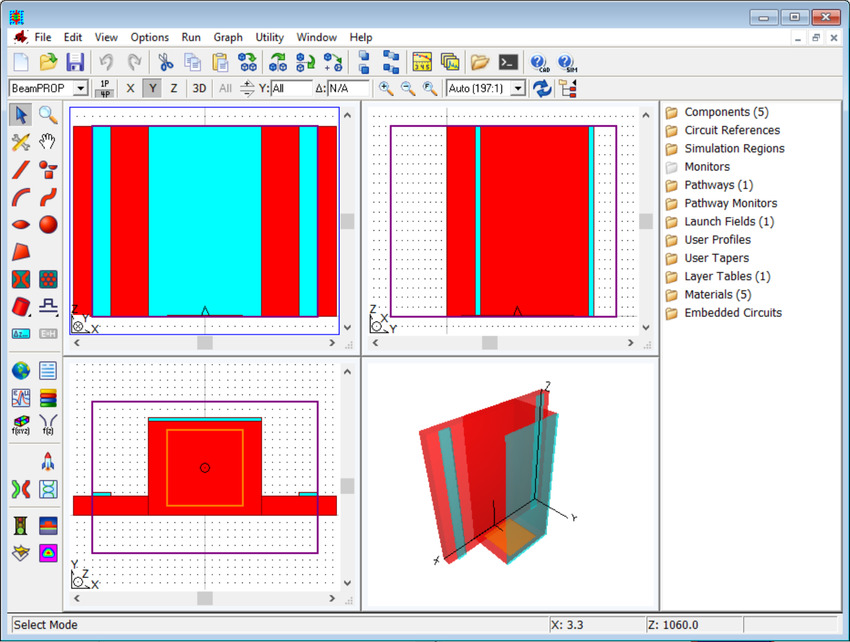Figure 1. CAD Layout of the AlGaAs ridge waveguide section (red segments) with negatively biased electrode (light blue segment) on top of the ridge, and ground electrodes on either side.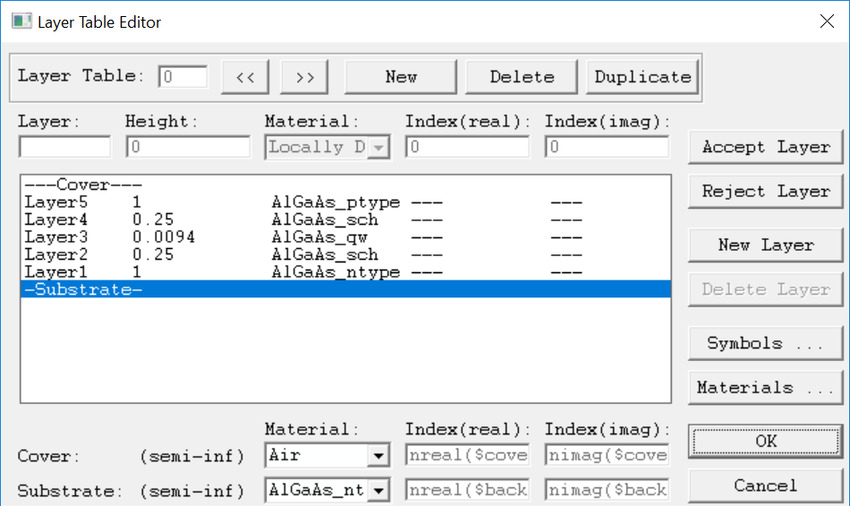Figure 2. Layer Table showing the epitaxial structure of the ridge waveguide. The off-ridge (or slab) will have the same layers but will be truncated by the slab-height. All heights are in μm.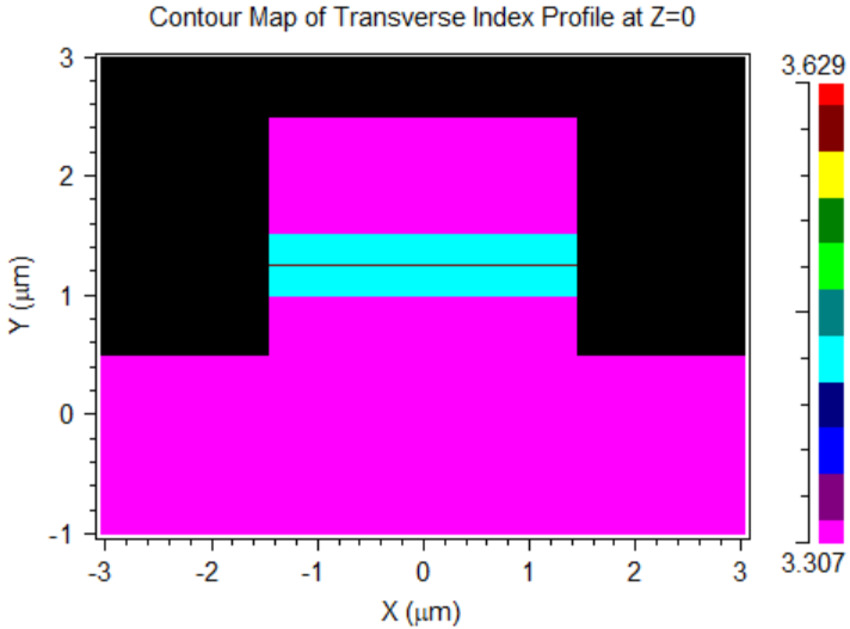Figure 3. Refractive index of the EAM at λ=0.852

When simulating exciton effects, the RSoft Multi-Physics Utility allows the user to control the number of valance and conduction subbands that will contribute to the exciton absorption. Formally, all transitions contribute, but we can limit the number to only the first few to reduce the computation time. These options default to 1, the number in practice will most likely be a problem-dependent choice. For this application note, we will set the # of valence subbands of the quantum well to 2, and the # of conduction subbands to 1.

## Running the Carrier-Optic Simulation

Running the Multi-Physics Utility from the dialog will generate the standard outputs associated with the carrier transport, such as C-V, R-V, and carrier density profiles, and also several additional plots showing the exciton absorption at various Stark fields, as well as the Stark field in the quantum well as a function of applied voltage.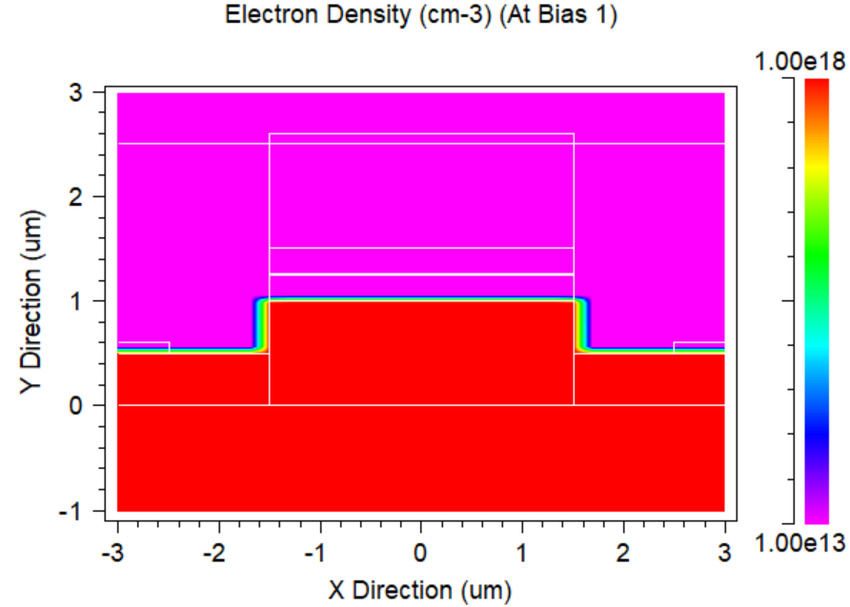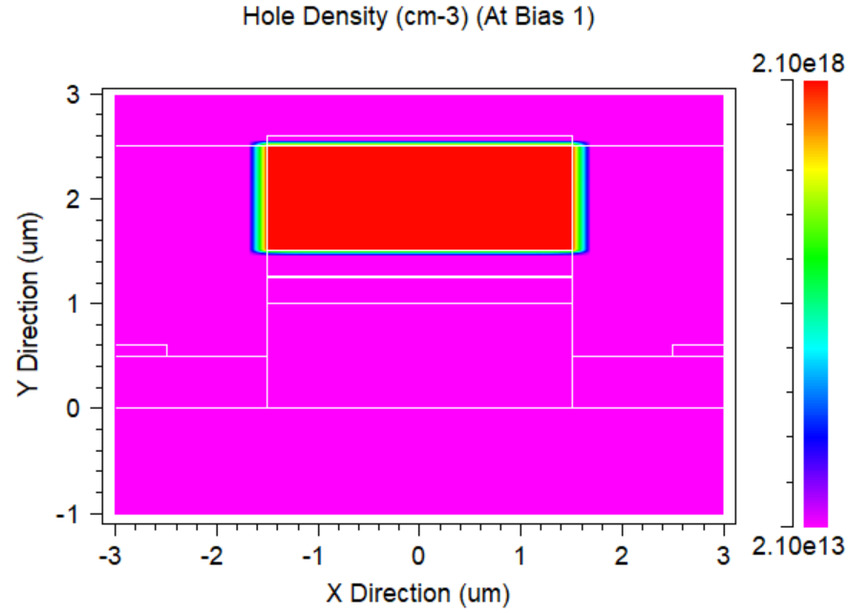Figure 4. Electron (left) and Hole (right) densities at -1V bias.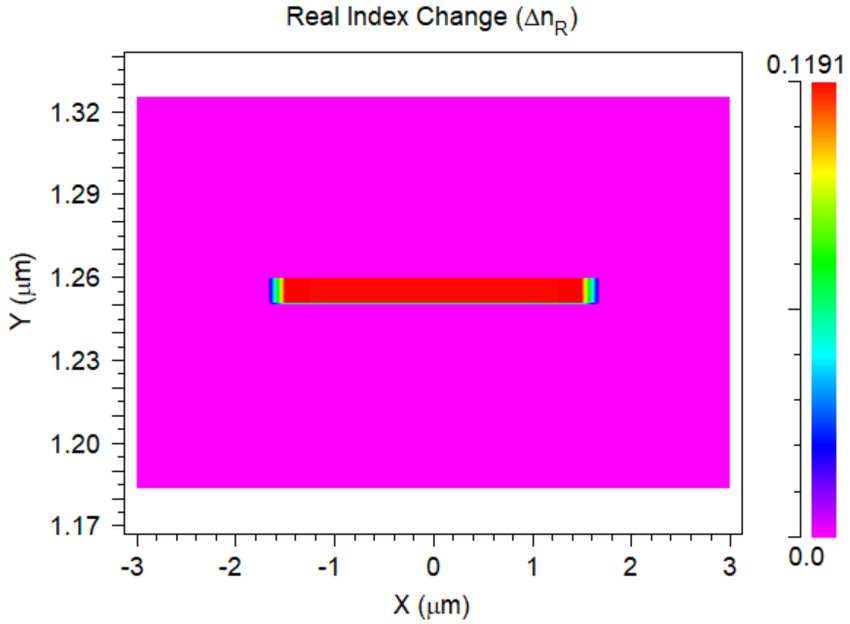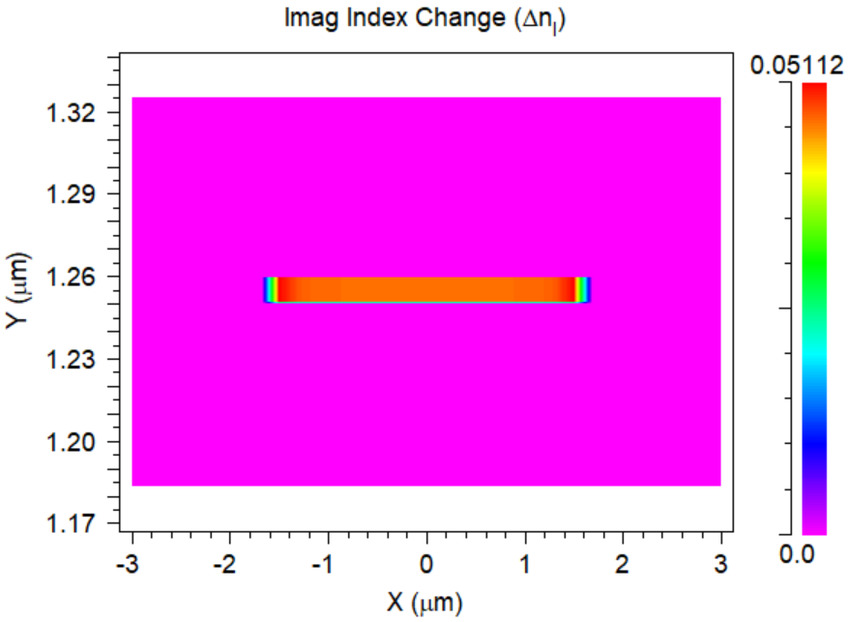Figure 5. Real (left) and Imaginary (right) index perturbations at -1V bias. These perturbations appear in the quantum well layer, which exists only in the ridge (figures are zoomed in near the well).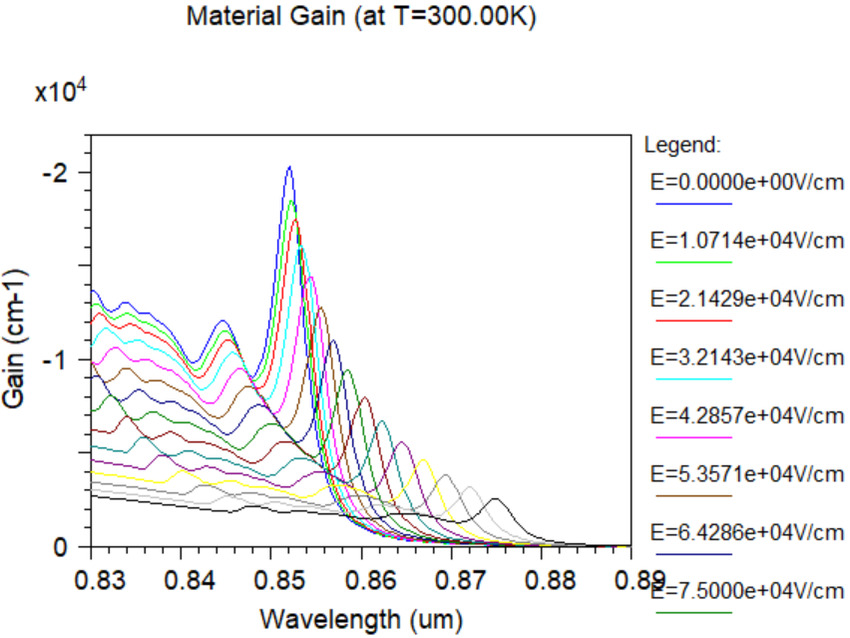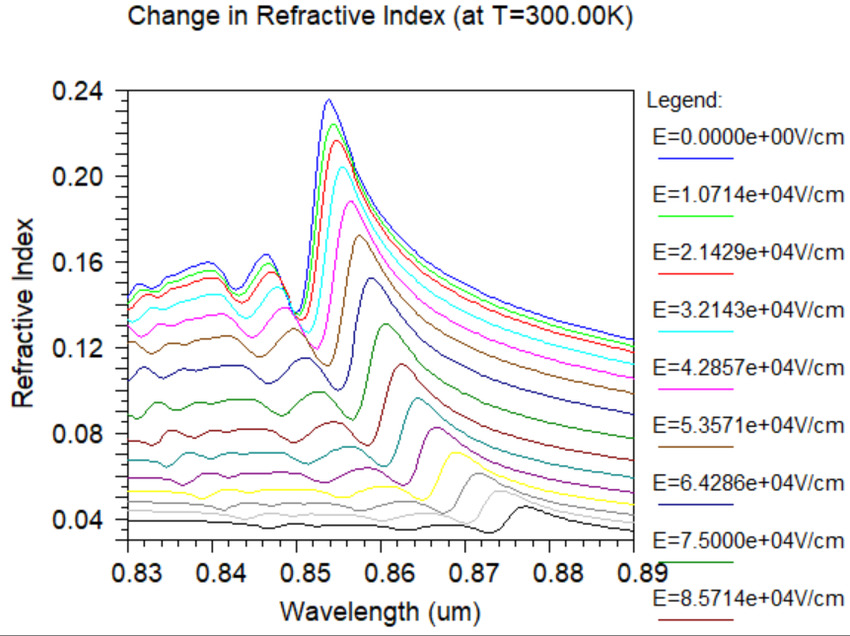Figure 6. Absorption (left) and index change (right) over the entire range of fields (0-150kV/cm by default). Note the redshift of the absorption peak with increasing field.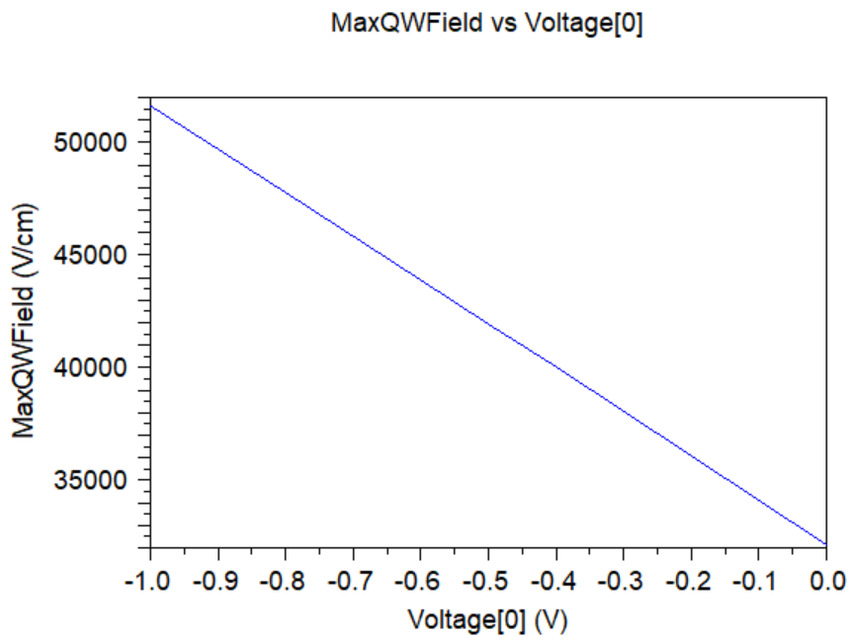Figure 7. Field values in the quantum well over the specified bias range (0 to -1V).

## Running the Optical Simulation

After computing the carrier dynamics, the RSoft mode solvers are then used to calculate the optical modes. Note that the transport simulation engine is automatically invoked by the mode solvers to determine the complex index perturbations, which will then be added to the refractive index/loss profiles of the circuit prior to each mode calculation.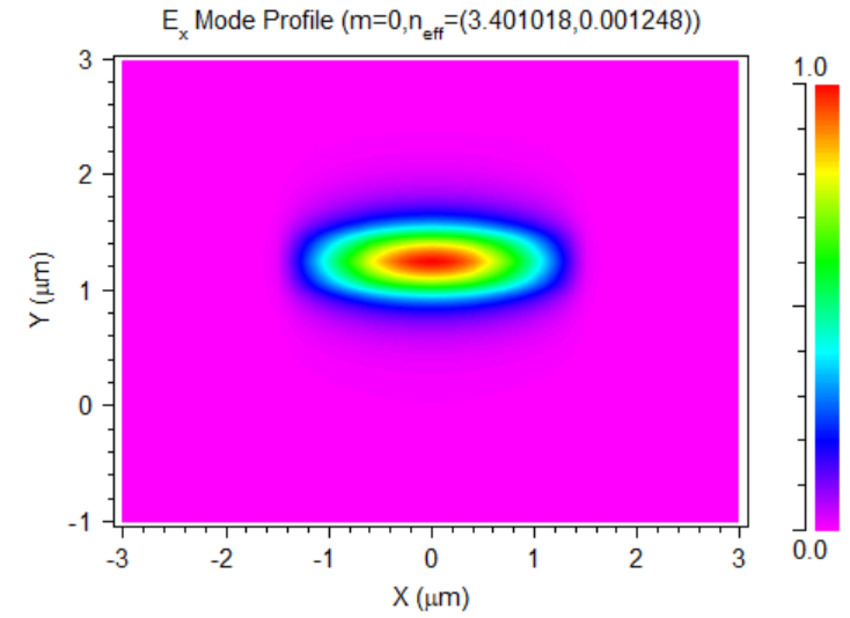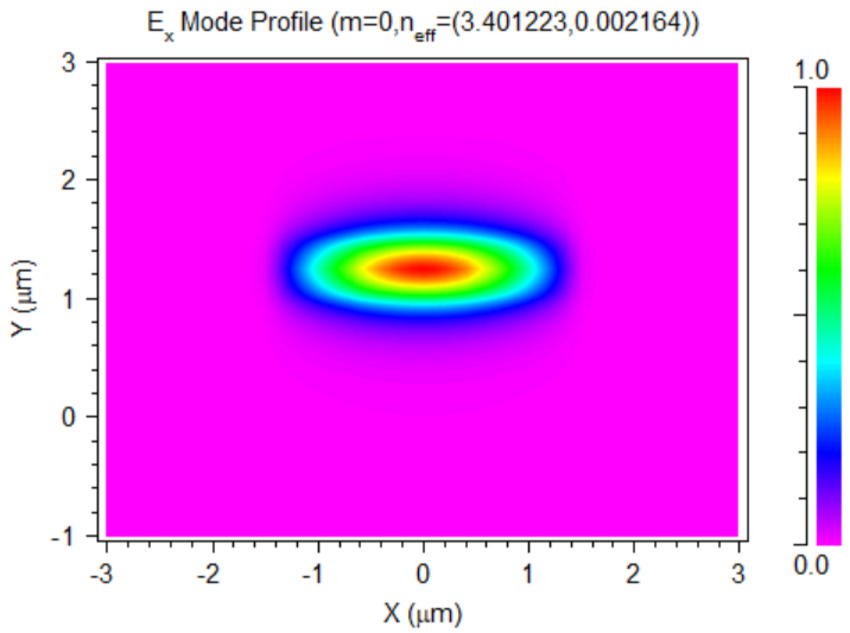Figure 8. TE mode at -1V (left) and -0.1V (right).

Finally, note that the results for 0V must include the carrier effects, especially the exciton effects, due to the built-in potential across the p-i-n junction, for accurate mode calculation.

The modulator performance, for a fixed length of waveguide, can be automatically calculated using the MOST multi-variable parameter scanning & optimization tool. The scan results are shown below.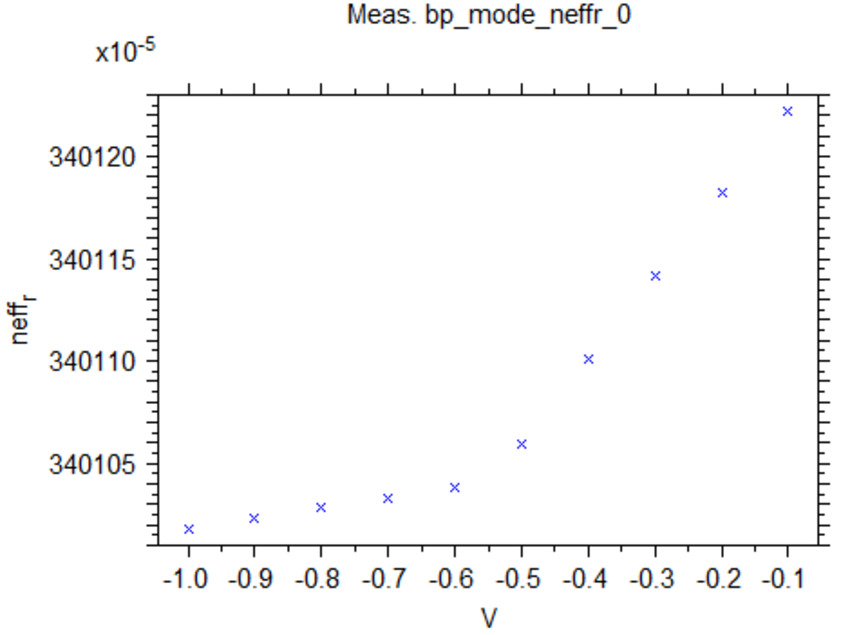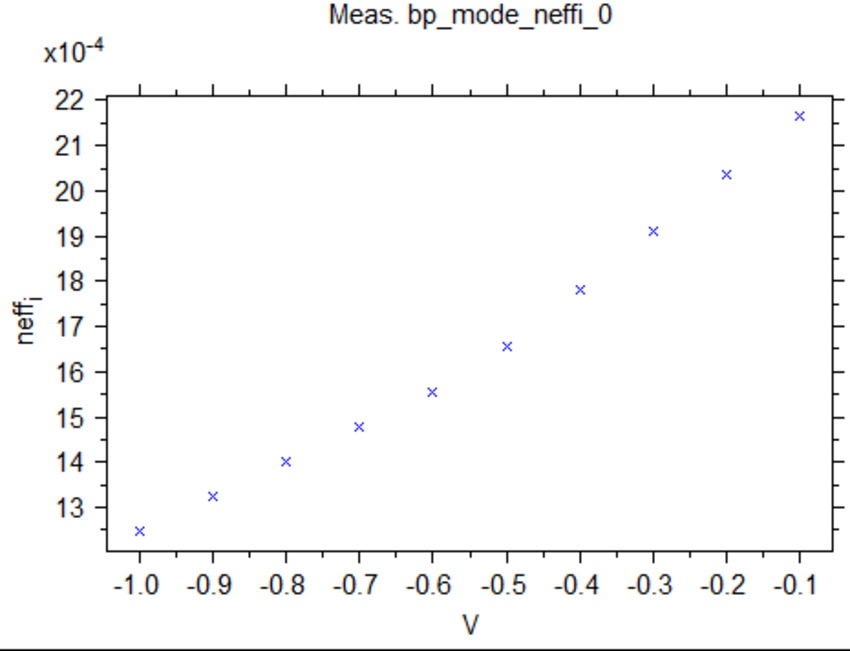Figure 9. MOST results showing the real (left) and imaginary (right) parts of the effective index as a function of applied voltage.